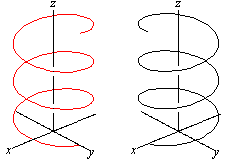# Is Parametric Equation for 2D and 3D a good solution

## Recommended Posts

From past few days I've been studying Electromagnetic Field Theory by Sadiku. As electromagnetic field theory requires knowledge of vector calculus so while studying I came to struck up with a problem which is related to parametric equation, which is also related to motion in one dimension to two and three dimensions.

So let me explain my doubt

Take a look at figure: Below is the graph for position vs timehere in above graph what we really show is that how a position of an object or particle varies with respect to time. In other words position is a function of time. so if "x" is used for position and "t" for time then x(t) = f(t); some function

Now have a look at below figure its a projectile motion (motion in two dimensions)and now for motion in three dimensions here are two figure shown belowIn all graph of two and three dimension one thing to be noted is that the graph is plotted for the position of any particle or object, but there is no variable for time.

but wait they have been parametrized as

in 2D

x = f(t) and y = g(t); in a similar way

in 3D

x = f(t); y = g(t) and z = h(t)

but in case of one dimension motion why it is so we've plotted with respect to time.

And for the case in 2 and 3D we haven't . Well for 3D it seems to be difficult as there isn't any axis for taking "t" as variable but in 2D what the problem.

So my problem is why we use parametric equation for 2 and 3D.

Edited by Ashish
##### Share on other sites

From the 2D and 3D plots, the motion can be understood rather well without a time coordinate. Try that with the 1D plot.

##### Share on other sites

From the 2D and 3D plots, the motion can be understood rather well without a time coordinate. Try that with the 1D plot.

thanks for showing interest but be little more comprehensive

##### Share on other sites

- What I said is that a plot without a time coordinate still carries some information in 2+ dimensions (look at the plots, they tell you something about what is happening, right?). In 1D, the respective plot is always just a line segment which carries little to no information. So no one plots line segments.

- I don't get your point about parametric equations. In 1D you describe a path as x(t), where x is a 1D vector. In 2D you describe a path as $\vec x(t)$ where $\vec x$ is a 2D vector (which also can be written as (x,y)(t) or (x(t),y(t)) or x(t), y(t) ). In 3D you describe a path as as $\vec x(t)$ where $\vec x$ is a 3D vector (which also can be written as (x,y,z)(t) or (x(t),y(t),z(t)) or x(t), y(t), z(t)). In other words: There's no difference, except for the dimensionality of the position vector.

Hope that's more clear and helpful.

##### Share on other sites

Yes timo I agree with you that in 1D motion there is little bit information.

Ok then have a look once more, the parametric equation of circle ( x^x + y^y = r^r) is given by

x = r cos(t)

y = r sin(t)

where parameter comes to be as an angle drawn by x-axis to radius measured in counter-clockwise direction. So that makes a sense that the variable t or parameter is there.

but in case with helix, projectile motion, or any 3D motion there isn't any variable or parameter that can be observed.

My problem is that why cant be embed them in one. or its just to show how the value of one variable varies.

or in other words in my views time should not be taken as another dimension as its always there.

Edited by Ashish
##### Share on other sites

I still don't really get your point. Perhaps this helps: The 2D and 3D plots without a time axis are more sensible than the 1D plot without a time axis, I think we agree there. They also give some information that might be helpful (e.g. in the 2D plot: how far did the stone fly till it came down again?). But they do not contain all of the information about the motion (e.g. not how long the stone flew). So they are not a complete graphical representation of $\vec x(t)$, but a helpful visualization.

##### Share on other sites

• 1 year later...

There are 3 length and 1 Time dimensions only.

FTOE

We can ignore other dimensions for our convenience but in reality there are 3 and 3 only length Ds and 1 time D. That's all, there is to it.

As there are only 3Ds, the 4thD can't be plotted. Time is an imaginary D. Imaginary in a sense, it has no physical existence. It is change... Time=Change. It can't be touched or eaten or smelled or felt or what ever... It does exist, it's change. We can plot a line - 1D, a 2D graph and a 3D graph but not more than 3D. You can't plot what can't exist.

Edited by way12go

## Create an account

Register a new account